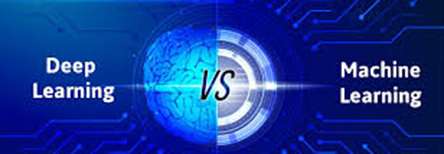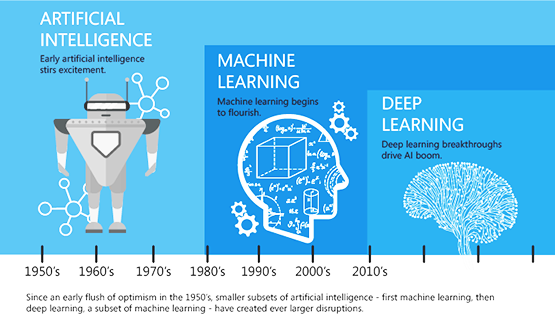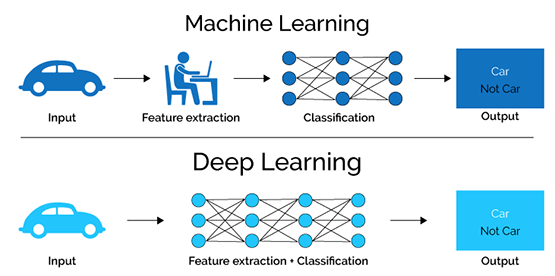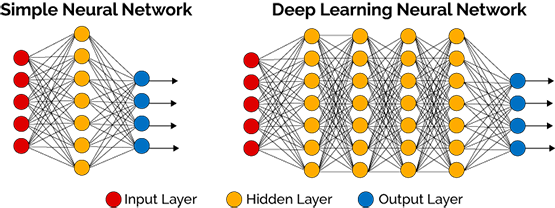## Deep Learning vs Machine-Learning## Deep Learning vs Machine-LearningDeep Learning vs Machine LearningMachine learning is that field of data science which consists of the algorithms that perform the learning procedure without human assistance.

Deep learning is the procedure used to implement machine learning, by the framework of the human brain. It is particularly efficient in feature detection.Perspective of Machine Learning vs Deep Learning

• Machine learning has various perspectives, ultimately expressed in ‘Artificial Neural Networks’ (ANN's).
• An ANN is a data processing system built on the structure and operation of biological neural networks.
• A distinct type of ANN which got a lot of attention and has seen significant progress is the Deep Learning NW.
• Thus, Deep Learning is a procedure practiced to implement machine learning.
• The nodes/neurons are positioned in layers: an input layer, an output layer, and several hidden layers.
• A neural network usually has 2 to 3 nodes while there are 100's of nodes in the Deep Learning NW.
• In the Deep Learning NW, each node is formed by mathematical functions that are used to describe how the nodes operate in the presence of an input signal.
• These input signals are derived from a digitized image, or sound/audio, etc.
• The responses of each node are combined, which results in a recognition of the objects in the digitized image or words in the sound/audio, that were applied at the output.
• Thus, the system learns, by developing a learning set of data. Normally, there is a set of inputs whose desirable outputs are already known.
• The mathematical functions of the nodes tune-up on their own, improving the system while performing.Main Differences between Machine vs Deep Learning

1. DATA DEPENDENCIES: The algorithms of deep learning operate much more effectively with Big Data bases.
2. HARDWARE DEPENDENCIES: Machine learning algorithms depend on low-end machines while deep learning algorithms depend on high-end machines, because deep learning algorithms include GPUs as an integral part of their operation.
3. PROBLEM-SOLVING APPROACH: With a machine learning algorithm, the problem is broken up in different levels. The problem is then solved per level. Then the solutions of each level are combined to form the solution of an entire problem. In deep learning the problem is solved end-to-end as a whole.
4. EXECUTION TIME: The many parameters inside the deep learning algorithms require a much longer training period compared to the fewer in machine learning. The reverse takes place at testing.
5. INTERPRETABILITY: Machine learning algorithms read clear rules, while deep learning rules are fuzzy.

Applications of Machine Learning and Deep Learning

• Medical Diagnosis: Both methods are used in medical imaging. The algorithms of machine learning are used in tumor detection and monitoring the development of a tumor, blood flow quantification and visualization etc.
• Digital Marketing: Both methods are used here, to optimize consumer engagement, with higher earnings as a result.
• Computer Vision: Both methods are used in this science, which deals with the automatic extraction of useful information from a large number of images in fields like robotics, transport, process control, etc.